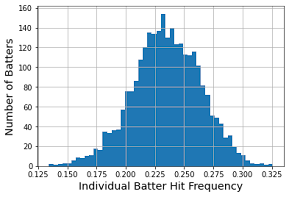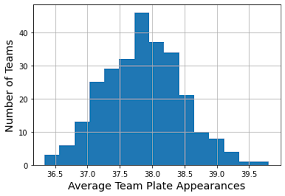### Beat the Streak: Day Eight

In this blog post, we will explore three factors that influence the probability of correctly selecting a player to get a hit on a given day.  These are:

1. Individual batter strength, as measured by the proportion of plate appearances that resulted in a hit.

2. Team offensive strength, as measured by the average number of plate appearances per game by the batting team.

3. The position in the batting order.

We plot the distribution of these statistics over (batter, year) pairs and (team, year) pairs.  The plots below reveal that the best batters get a hit in about 30% of plate appearances, and the strongest offensive teams average 39 plate appearances per game.The tables below show the top-performing batters and teams:

batter year
Josh Hamilton 2010 0.326
Trea Turner 2016 0.324
Jose Altuve 2014 0.319
Daniel Murphy 2016 0.316
Melky Cabrera 2012 0.315
Dee Gordon 2015 0.315
Hanley Ramirez 2013 0.312
Michael Young 2011 0.310
Carlos Gonzalez 2010 0.310
batter_team year
Mets 2011 39.000
Yankees 2017 39.006
Braves 2018 39.051
Red Sox 2010 39.093
Reds 2018 39.143
Red Sox 2013 39.253
Yankees 2010 39.253
Tigers 2013 39.278
Red Sox 2011 39.389

Note that the data I am working with currently covers years 2010 - 2018, which is somewhat stale, but should be sufficient for the purposes of understanding these general factors.  For an individual team, we can effectively model the number of plate appearances with a negative binomial distribution.  This is a distribution that counts the number of successes (i.e., non-outs) before a pre-specified number of failures (i.e., outs).  We set the number of failures, $r$ to be $27$.  Note that this model is simply an approximation to the true distribution.  In home games, $24$ outs might be sufficient, and in extra-innings games more would be needed.  Moreover, this model does not account for the possibility of double and triple plays, nor the fact that different players in the lineup have difference chances of success.  Nevertheless, it serves as a simple and reasonable model for this quantity.  We can fit the model to data using the moment matching method.  Doing this on the 2016 Red Sox data yields the following plot:

This demonstrates that the fit is reasonable.  Now, we will analytically try to determine the probability that an individual player will get a hit as a function of the three factors mentioned at the beginning of this post.  Let's assume that each player has a unique single-plate-appearance hit probability, which we will denote $p$.  Now if that players has $n$ plate appearances, the probability that they will get a hit in any one plate appearance is simply $1 - (1 - p)^n$.  However, the number of plate appearances for an individual player is a random quantity that depends on the number of plate appearances for the team.  Let $N$ denote the number of plate appearances for the team, which we assumed was sampled from a negative binomial distribution.  Then we have:

$$n = \lfloor 1 + \frac{N - order}{9} \rfloor$$

Here, "order" is the position in the lineup of the player.  We can calculate the probability of each $n$ using the probability mass function of the negative binomial, to estimate the overall probability of a hit:

$$\sum_{N=27}^{\infty} f(N) (1 - (1 - p)^{ \lfloor 1 + \frac{N - order}{9} \rfloor })$$

$f(N)$ above denotes the PMF of the negative binomial.  Plotting this function for a set of reasonable inputs yields the following contour plot:

This plot was constructed under the assumption of a lead-off batter.  The color indicates the probability of at least one hit in the game, whereas the x-axis indicates the probability of a hit in a single plate appearance.  This plot shows that, roughly speaking, picking a batter with 1% better chance of a hit in one plate appearance is equivalent to picking a team that gets 2 more plate appearances on average.  This plot was for the lead-off batter.  The plot below shows what the probability of a hit is for batters in different positions in the lineup, assuming $0.3$ hit probability per plate appearances and an average of 38 team plate appearances under the negative binomial model.  This plot demonstrates that, all else equal, each position in the lineup costs about 0.8% to the probability of at least one hit.

This blog post suggests that an 80% success rate in BTS should be attainable, by carefully considering the batter, the team, and the position in the lineup.  For some reason that number has been elusive to me so far in my efforts to beat the streak.  Even though as we saw above that some hitters were able to get hits in 30% of plate appearances in a given season, it's entirely possible that this impressive success rate was due in part by luck, and the true success rate (which is unknown) could be below 30%.  This could partially explain why an 80% success rate has been so hard to achieve in BTS.